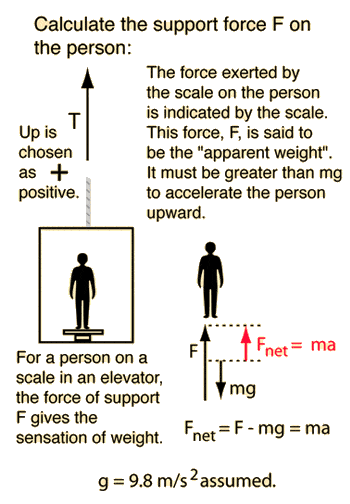# Can someone help me with The Normal Force

Can someone help me with "The Normal Force"

1) Anyone know how to work out these problems?

A 42 kg crate rests on a horizontal floor, and a 76 kg person is standing on the crate.
(a) Determine the magnitude of the normal force that the floor exerts on the crate.

(b) Determine the magnitude of the normal force that the crate exerts on the person.

2) A 86.0 kg person stands on a scale in an elevator. What is the apparent weight in each of the following situations?
(a) when the elevator is accelerating upward with an acceleration of 1.10 m/s2

(b) when the elevator is moving upward at a constant speed

(c) when the elevator is accelerating downward with an acceleration of 1.20 m/s2

3) A car is traveling up a hill that is inclined at an angle of above the horizontal.

Determine the ratio of the magnitude of the normal force to the weight of the car when = 12° Whats the Ratio?

Determine the ratio of the magnitude of the normal force to the weight of the car when = 32°Whats the Ratio?

If this is homework it is supposed to be posted in the homework threads.

Have you tried solving these problems? What have you got so far? I will try help you from where you are at. = D

i am just giving these hints for a better understanding of the prob..do not copy the anser without learning the basic concept...:

1.a.normal force by floor on crate=42*9.8+76*9.8 N
b.normal force by crate on the person=76*9.8

The working of above solutions has been done using newton's third law.

2.Elevator ProblemThis is an application of Newton's second law to the forces felt in an elevator. If you are accelerating upward you feel heavier, and if you are accelerating downward you feel lighter. If the elevator cable broke, you would feel weightless since both you and the elevator would be accelerating downward at the same rate.
support force F = mass x acceleration + weight

For a mass m= kg, the elevator must support its weight = mg =Newtons to hold it up at rest. If the acceleration is a=m/s² then a net force=Newtons is required to accelerate the mass. This requires a support force of F=Newtons. Note that the support force is equal to the weight only if the acceleration is zero, and that if the acceleration is negative (downward), the support force is less than the weight. If you enter a downward acceleration greater than 9.8 m/s² you will get a negative support force, showing that you must force it downward to get an acceleration greater than that of free fall.

3.Normal Force
* The normal force on a body is generally associated with the force that the surface of one body exerts on the surface of another body in the absence of any frictional forces between the two surfaces.

* The normal force is always perpendicular to the surfaces in contact. This is the origin of its name - normal to the surface.

* The normal force is an action-reaction force. A surface will not exert a normal force on an object in contact with it unless some other external force pushes the object into the surface.

* The atoms in the surface are compressed microscopically to create the normal force. The surface deforms imperceptibly and produces a reaction force equal to the force pressing the object into the surface.

* For an object sitting on a horizontal surface, the normal force will be equal to the weight of the object.

* The normal force is not always equal to the weight of an object; it is the force pressing the surfaces together.

http://www.ac.wwu.edu/~vawter/PhysicsNet/Topics/Dynamics/gifs/Forces10.gif [Broken]

[PLAIN]http://www.ac.wwu.edu/~vawter/PhysicsNet/Topics/Dynamics/gifs/Forces11.gif [Broken]

[PLAIN]http://www.ac.wwu.edu/~vawter/PhysicsNet/Topics/Dynamics/gifs/Forces11.gif [Broken]

#### Attachments

Last edited by a moderator:
ok so i understand in order to get the normal force u have to include gravity=9.8...

for question 2) would i use ∑F=ma...but i don't understand the part about constant speed?

And for number 3...i start by adding 90 degree + 12 degrees= 102, then 180-102=78 then i got lost when it asked for the ratio.

PhanthomJay
Homework Helper
Gold Member
for question 2) would i use ∑F=ma...
yes
but i don't understand the part about constant speed?
If the speed is constant, what is the acceleration?
And for number 3...i start by adding 90 degree + 12 degrees= 102, then 180-102=78 then i got lost when it asked for the ratio.
You should draw the x axis parallel to the slope of the inclined plane, and the y axis perpendicular to the slope of the plane. In this coordinate system, the normal force will act along the y axis, as 'physixguru' has pointed out. Now use some trig and geometry and calculate the normal force as a function of the weight by using Newton 1 in the y direction, because there is no acceleration in the y direction when you choose your axes this way.

Last edited:

* The normal force is an action-reaction force. A surface will not exert a normal force on an object in contact with it unless some other external force pushes the object into the surface.

Sorry for bumping an old thread :shy:

I am learning about newton's third law and my textbook says that the normal force is not an action-reaction force. I am confusedPhanthomJay
I am learning about newton's third law and my textbook says that the normal force is not an action-reaction force. I am confused